﻿ 跳扩散下汇率变动的外商直接投资问题研究文章快速检索 高级检索

Study of a foreign investor's investment with fluctuations of exchange rate under jump-diffusion
, HE Dan-dan, ZHANG Wei
Department of Financial Engineering, Anhui Polytechnic University, Wuhu 241000, China
Abstract:This paper studies a foreign investor's direct investment with fluctuations of exchange rate under jump-diffusion environment. First, we obtain the dynamics of asset price denoted by native currency by using Itô formula. Then, under maximizing the expected utility of the terminal wealth, through using HJB equation, we obtain the optimal allocation strategy, and derive an approximate solution of the optimal dynamic asset allocation. Finally, we analyze the impacts of the jump and the fluctuations of exchange rate on the optimal allocation strategy of an investor through a numerical simulation.
Key words: jump-diffusion process     fluctuations of exchange rate     optimal portfolio     HJB equation     stochastic calculus

0 引言

 $\frac{{ d}H(t)}{H(t)}=h{ d}t+\delta { d}Z(t),H(0)=H_0$ (1)

 $\frac{{ d}P_t}{P_t}=(\mu_t-\lambda g){ d}t+\sigma { d}B(t)+({ e}^q-1){ d}Q(\lambda)$ (2)

 ${ Pr}(\tau \mbox{期限内$n$个跳})={ e}^{-\lambda\tau}\frac{(\lambda \tau)^n}{n!}$ (3)

 $\begin{array}{rll} { d}\hat{P}(t)&={ d}(H(t)P(t)) =H(t){ d}P(t)+P(t){ d}H(t)+{ d}H(t)P(t)\\ &=\hat{P}(t)(\mu_t-\lambda g+h+\delta\sigma\rho)+\hat{P}(\delta { d}Z(t)+\sigma { d}B(t))+\hat{P}(t)({ e}^q-1){ d}Q(\lambda)\\ &=\hat{P}(t)(\mu_t-\lambda g+h+\delta\sigma\rho)+\hat{P}(t)\sqrt{\sigma^2+\delta^2+2\delta\sigma\rho}{ d}\hat{B}(t)+\hat{P}(t)({ e}^q-1){ d}Q(\lambda), \end{array}$

 ${ d}P_0=rP_0{ d}t$ (4)

 $\eta_t=\frac{\mu_t-\lambda g+h+\delta\sigma\rho-r}{\sqrt{\sigma^2+\delta^2+2\delta\sigma\rho}}$ (5)

 $\begin{array}{rll} { d}W_t&=(1-\theta_t)W_t r{ d}t+\theta_tW_t[(\mu_t-\lambda g+h+\delta\sigma\rho-r){ d}t+\hat{\sigma}{ d}\hat{B}(t)+({ e}^q-1){ d}Q(\lambda)]\\ &=rW_t { d}t+\theta_tW_t[\hat\sigma\eta_t+\hat\sigma { d}\hat{B}(t)+({ e}^q-1){ d}Q(\lambda)] \end{array}$ (6)

 $J(W,H,\tau)=J(W_t,H_t,\tau)=\max\limits_{\theta_t}E_t[{ e}^{-r\tau}U(W_T)]$ (7)

 $\begin{array}{rll} 0=&\max\limits_{\theta_t}\bigg\{-J_\tau+\lambda E_t[J(W',H,\tau)-J(W,H,\tau)]+J_W rW+J_W \theta\hat{\sigma}\eta W+\\ &\frac{1}{2}J_{WW}\theta^2\hat{\sigma}^2W^2+J_H h+\frac{1}{2}J_{HH}\delta^2+J_{WH}\theta \hat{\sigma}\delta\hat{\rho}W\bigg\} \end{array}$ (8)

 $E[{ d}\hat{B}(t)Z(t)]=\hat{\rho}{ d}t=\frac{\delta+\sigma\rho}{\sqrt{\sigma^2+\delta^2+2\delta\sigma\rho}}$ (9)
(8)式中的$W'_t=W_t[1+\theta_t({ e}^q-1)]$是一个跳发生下的财富水平,当完全违约或破产的特殊情形时(即$q=-\infty$),投资者的财富减少到$W'_t=W_t[1-\theta_t]$. 假设投资者的效用函数满足$U'(0)=\infty$,$U'(\infty)=0$,则为了保证投资者的财富为正值,投资者不会将所有的资产全部配置在风险资产中,即在任何时候$\theta_t<100\%$.

 $\theta^*(W,H,\tau)=\bigg(\frac{-J_W}{J_{WW} W_t}\bigg)\frac{\eta_t}{\hat{\sigma}}+\bigg(\frac{-J_{WH}}{J_{WW} W_t}\bigg)\frac{\delta\hat{\rho}} {\hat{\sigma}}+\frac{\lambda E_t[J_W'(W',H,\tau)({ e}^q-1)]}{-J_{WW} W\hat{\sigma}^2}$ (10)

 $U(W)=\frac{W^{1-\alpha}}{1-\alpha},\alpha>0$ (11)

 $J(W,H,\tau)\approx\Phi(H,\tau)U({ e}^{r\tau}W)$ (12)

 $\Phi(H,\tau)=\mathrm{exp}(A(\tau)+B(\tau)H+C(\tau)H^2 /2)$ (13)

 $\frac{{ d}C}{{ d}\tau}=aC^2,\frac{{ d}B}{{ d}\tau}=aBC+bC,\frac{{ d}A}{{ d}\tau}=\frac{a}{2}B^2+(b+c\hat{\rho}\delta\eta_t)B+\frac{1}{2}\delta^2C+D_t$ (14)

 $\theta^*(W,H,\tau)=\frac{\eta_t\hat{\sigma}+\lambda\hat{g}_t+\hat{\sigma}\delta\hat{\rho}[B(\tau)+C(\tau)H]} {\alpha\hat{\sigma}^2}$ (15)

 $C(\tau)=-\frac{1}{a(\tau+1)},\ \ B(\tau)=-\frac{1-b(\tau+1)}{a(\tau+1)}$ (16)
1. 2 模型结论

 $\theta^*(W,H,\tau)=\frac{\eta_t\hat{\sigma}+\lambda\hat{g}_t+\hat{\sigma}\delta\hat{\rho}[B(\tau)+C(\tau)H]} {\alpha\hat{\sigma}^2},$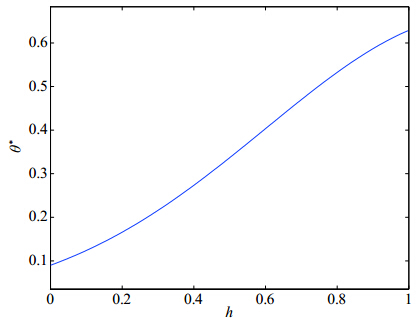图 1 即期预期汇率$h$对最优投资组合$\theta^*$的影响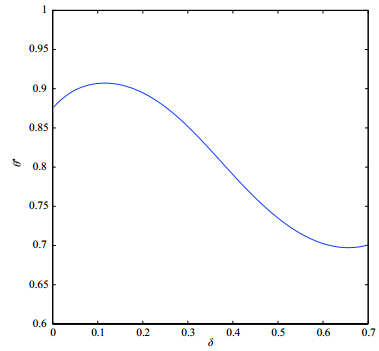图 2 汇率波动率$\delta$对最优投资组合$\theta^*$的影响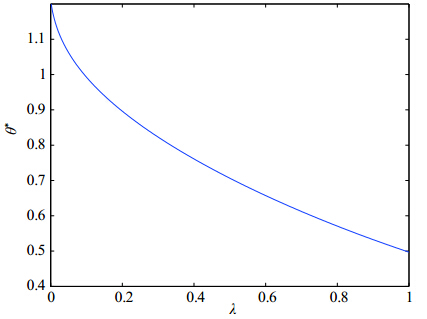图 3 跳强度$\lambda$对最优投资组合$\theta^*$的影响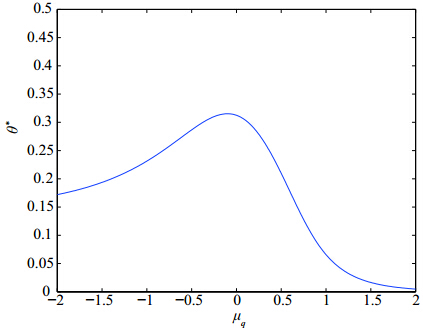图 4 跳大小$\mu_q$对最优投资组合$\theta^*$的影响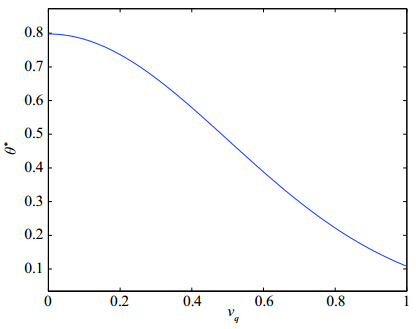图 5 跳波动率$\nu_q$对最优投资组合$\theta^*$的影响

  Press S J. A compound events model for security prices[J]. Journal of Business, 1967, 40(3): 317-335.  Merton R C. Optimum consumption and portfolio rules in a continuous-time model[J]. Journal of Economic Theory, 1971, 3(4): 373-413.  Yacine A S, Julio C D, Hurd T R. Portfolio choice with jumps: A closed-formd solution[J]. The Annals of Applied Probability, 2009, 19(2): 556-584.  Eraker B. Do stock prices and volatility jump? Reconciling evidence from spot and option prices[J]. Journal of Finance, 2004, 59(3): 1367-1403.  Das D, Uppal R. Systemic risk and international portfolio choice[J]. Journal of Finance, 2004, 59(6): 2809-2834.  Jin X, Zhang A. Ambiguity aversion, optimal portfolio choice and market decomposition with rare events[EB/OL]. Working Paper, 2011[2013-05-09]. www.ccfr.org.cn/cicf2011/papers/20110523013543.pdf.  Hanson F B. Stochastic-volatility, jump-diffusion optimal portfolio problem with jumps in returns and volatility[EB/OL]. Working Paper, 2011[2013-05-10]. http://homepages.math.uic.edu/ hanson/pub/SIAMCT11/SIAMCCA2011ytde.pdf.  Callegaro G, Vargiolu T. Optimal portfolio for HARA utility functions in a pure jump multidimensional incomplete market[J]. Risk Assessment and Management, 2009, 11(2): 180-200.  Fei W Y, Wu R Q. A study on the model of the international investors' portfolio investment decision[J]. Mathematics in Economics, 1999, 16(2): 39-46.  Kim T S, Omberg E. Dynamic nonmyopic portfolio behavior[J]. Review of Financial Studies, 1996, 9(1): 141-161.  郭文旌, 赵成国, 袁建辉. 跳跃扩散市场的最优保险投资决策[J]. 系统工程理论与实践, 2011, 31(4): 749-760.Guo Wenjing, Zhao Chengguo, Yuan Jianhui. Optimal investment decision for insurer in a jump-diffusion market[J]. Systems Engineering —— Theory & Practice, 2011, 31(4): 749-760.  邓国和, 杨向群. 有跳风险的随机利率与动态资产分配[J]. 高校应用数学学报A辑, 2006, 21(3): 278-284.Deng Guohe, Yang Xiangqun. Dynamic asset allocation with stochastic interest rates in jump-risk[J]. Applied Mathematics Journal of Chinese University Series A, 2006, 21(3): 278-284.  Wu L R. Jump and dynamic asset allocation[J]. Review of Quantitative Finance and Accounting, 2003, 20(3): 207-243.  费为银, 蔡振球, 夏登峰. 跳扩散环境下带通胀的最优动态资产配置[J]. 管理科学学报, 即将发表.Fei Weiyin, Cai Zhenqiu, Xia Dengfeng. Dynamic asset allocation with inflation under jump-diffusion environment[J]. Journal of Management Sciences, to appear.  Kozo K, Shujiro U. Exchange rate, exchange rate volatility and foreign direct investment[J]. The World Economy, 2004, 27(10): 1501-1536.  Wheeler M, Chowdhury A R. Does real exchange rate volatility affect foreign direct investment? Evidence from four developed economies[J]. The International Trade Journal, 2008, 22(2): 218-245.  Chen K M, Rau H H, Lin C C. The impact of exchange rate movements on foreign direct investment: Market-oriented versus cost oriented[J]. The Developing Economies, 2006, 44(3): 269-287.  Rajeshv C, Bany S. Exchange rate expectations and foreign direct investment flows[R]. Working Paper, University of Alberta, 2002.  张静, 汪寿阳. 人民币均衡汇率与中国外贸[M]. 北京: 高等教育出版社, 2005.Zhang Jing, Wang Shouyang. Equilibrium exchange rate of RMB and the foreign trade of China[M]. Beijing: Higher Education Press, 2005.  孙宵, 刘士余, 宋逢明. 汇率调整对外商直接投资的影响[J]. 数量经济技术经济研究, 2006(8): 68-77.Sun Xiaochong, Liu Shiyu, Song Fengming. Effect of exchange rate adjustment and its impact on foreign direct investment[J]. The Journal of Quantitative and Technical Economics, 2006(8): 68-77.  闫伟, 李树荣. 带有汇率因素的不连续价格过程的最优投资组合研究[J]. 运筹与管理, 2008, 17(3): 134-139.Yan Wei, Li Shurong. Optimal portfolio with exchange rate based on discontinuous price process[J]. Operations Research and Management Science, 2008, 17(3): 134-139.  程瑶, 于津平. 人民币汇率波动对外商直接投资影响的实证分析[J]. 世界经济研究, 2009(3): 75-82.Cheng Yao, Yu Jinping. RMB exchange rate fluctuations on foreign direct investment empirical analysis[J]. World Economy Study, 2009(3): 75-82.  彭红枫. 汇率对FDI的影响:基于实物期权的理论分析与中国的实证[J]. 中国管理科学, 2011, 19(4): 60-67.Peng Hongfeng. The impact of exchange rate on FDI: The theory analysis based on real option and empirical study in China[J]. Chinese Journal of Management, 2011, 19(4): 60-67.  Mark P T, David A P, Lucio S. Non-linear mean reversion in real exchange rates: Towards a solution to the purchasing power parity puzzles[J]. International Economic Review, 2001, 42(4): 1015-1042.  王璐. 汇率均值回复的非线性STAR模型[J]. 统计与信息论坛, 2007, 22(4): 50-53.Wang Lu. Nonlinear STAR model of exchange rate in mean reverting[J]. Statistics and Information Forum, 2007, 22(4): 50-53.  张志柏. 人民币对美元实际汇率的均值回复研究[J]. 商业经济, 2012(4): 55-57.Zhang Zhibai. The research of mean reversion of the real exchange rate on RMB convert U.S. dollar[J]. Business Economy, 2012(4): 55-57.  Fei W Y. Optimal consumption and portfolio under inflation and Markovian switching[J]. Stochastics: An International Journal of Probability and Stochastic Processes, 2013, 85(2): 272-285.
0

#### 文章信息

FEI Wei-yin, HE Dan-dan, ZHANG Wei

Study of a foreign investor's investment with fluctuations of exchange rate under jump-diffusion

Systems Engineering - Theory & practice, 2015, 35(2): 283-290.